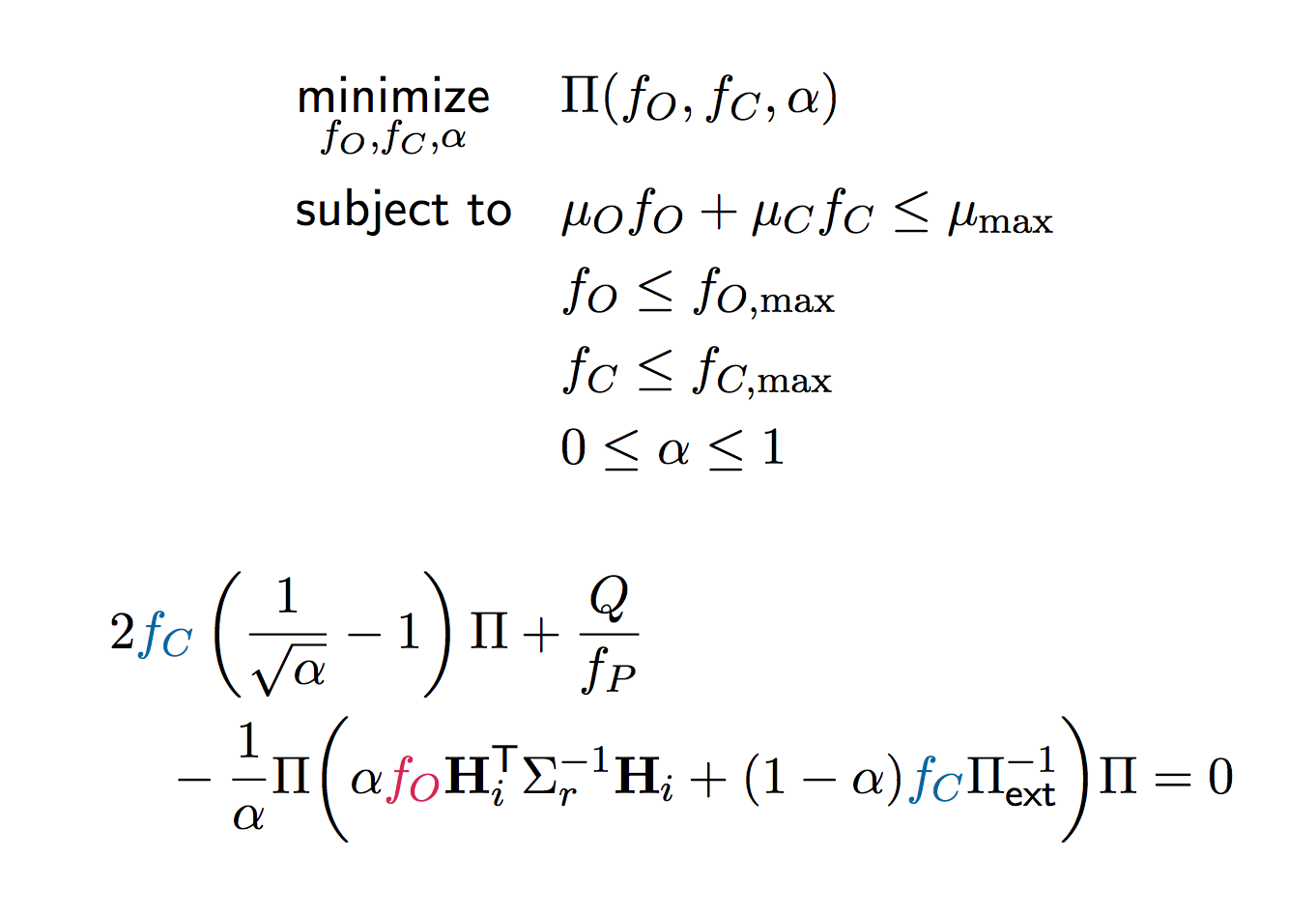08 Aug

#### Weekly Update: Solving the Optimization Problem

[[ Internal only ]]After formulating the continuous-time version of the Riccati recursion, it is time to solve the corresponding optimization problem. However, this problem turned out to be not easy at all.

First of all, the convexity of a matrix function should be clarified. While the parameters are scattered in several terms in the equation, I haven't got an idea how to solve it.

I tried CVXPY, which is a convex optimization solver. But the solver is not applicable in this case. The solver required a disciplined expression of constraints. However, in our problem, the relation is implicit.

I also found that there is a CARE (continuous-time algebraic Riccati equation) solver in MATLAB, but it is only available in the later version of MATLAB.

One possible way is to comprehend semidefinite programming. Maybe it will shed some light.